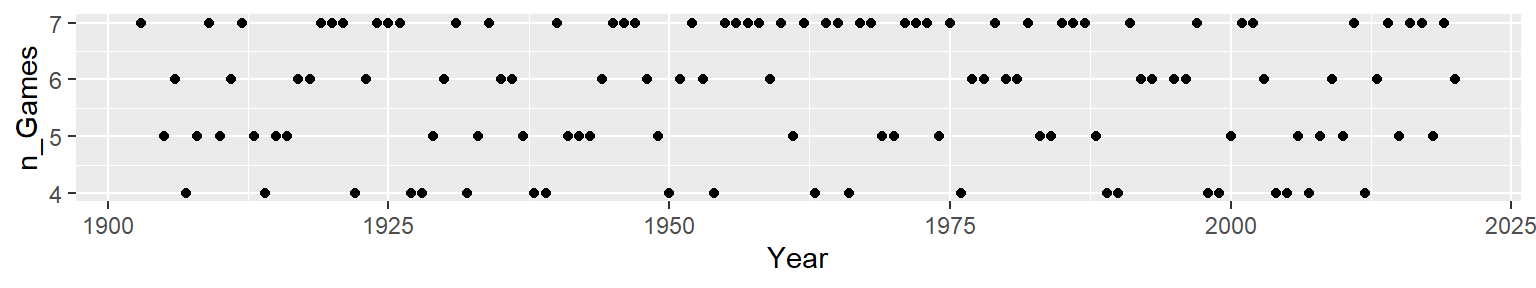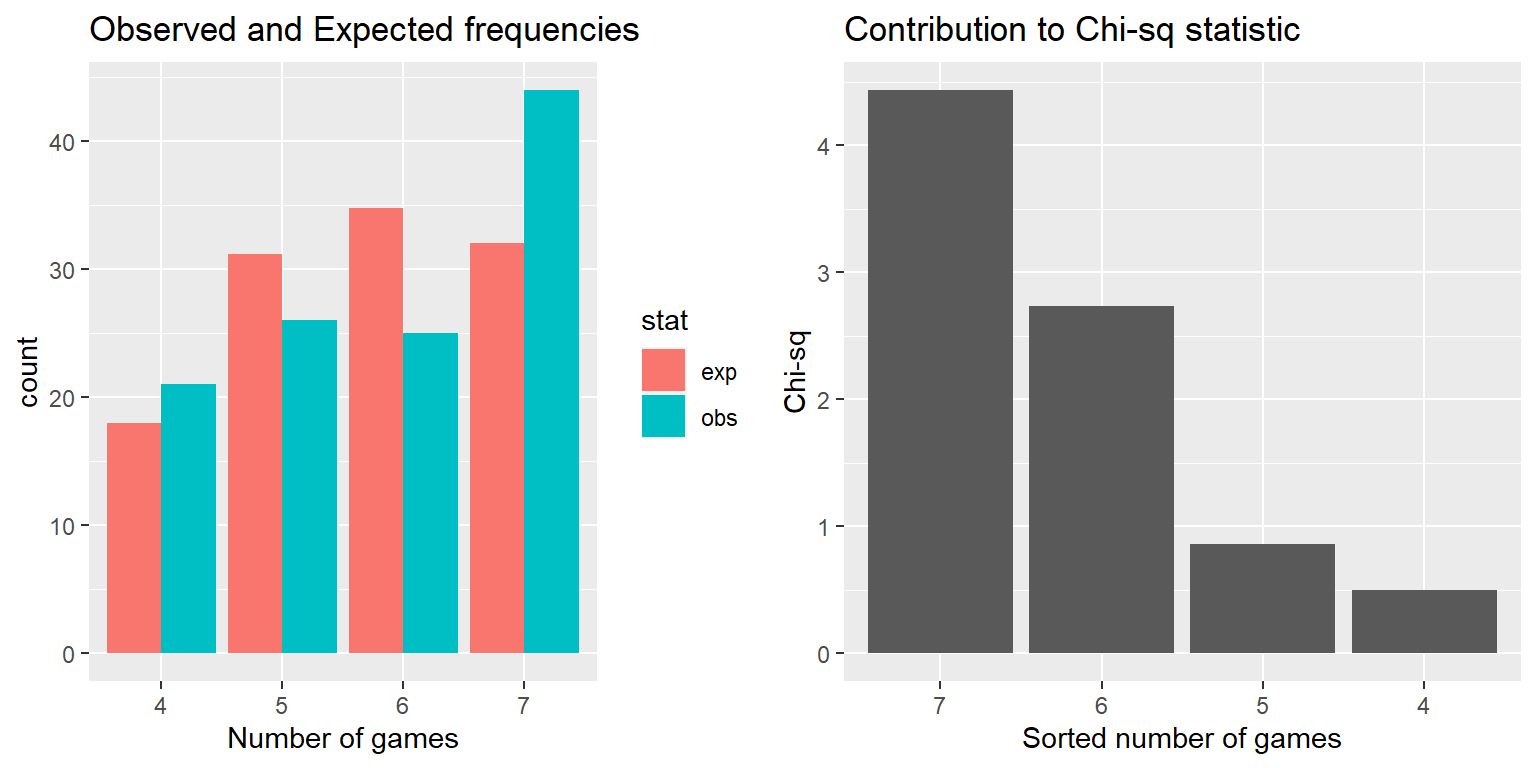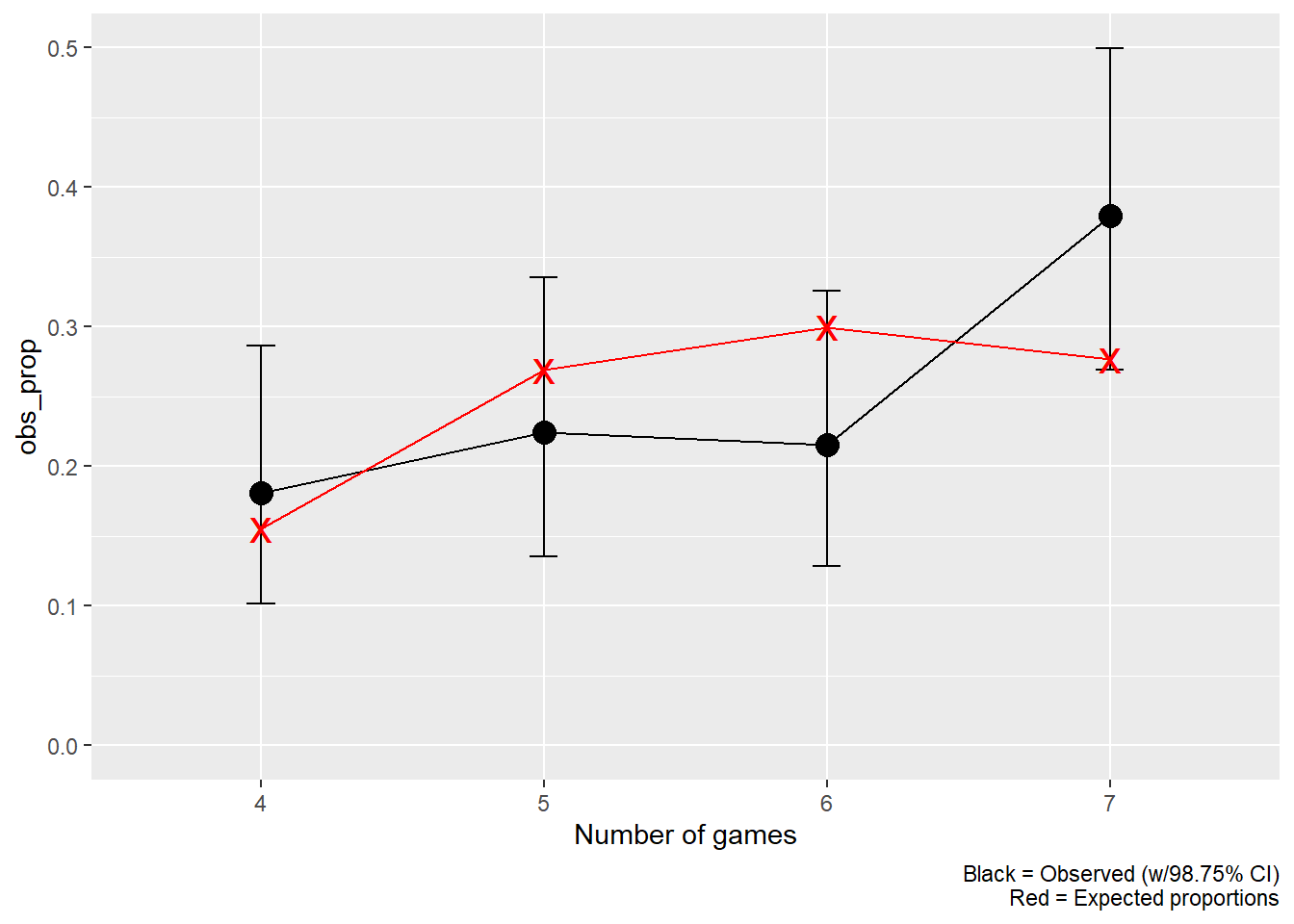# ADA1: Class 24, Binomial and Multinomial tests

Advanced Data Analysis 1, Stat 427/527, Fall 2022, Prof. Erik Erhardt, UNM

Author

Published

August 13, 2022

Include your answers in this document in the sections below the rubric where I have point values indicated (1 p).

# Rubric

Answer the questions with the data example.

# World Series expected number of games

One form of sportsball is the game of Baseball. Its championship, played exclusively in North America, is called the “World Series”.

How well does the distribution of number of games played during the Baseball World Series follow the expected distribution? This is a multinomial goodness-of-fit test. There are four categories for the number of games (4, 5, 6, and 7) and for each World Series the number of games must fall into one of these categories.

## Calculate observed and expected frequencies

We read in a table summarizing the World Series history, and calculate the total number of games played.

library(tidyverse)
── Attaching packages ─────────────────────────────────────── tidyverse 1.3.2 ──
✔ ggplot2 3.3.6     ✔ purrr   0.3.4
✔ tibble  3.1.8     ✔ dplyr   1.0.9
✔ tidyr   1.2.0     ✔ stringr 1.4.0
✔ readr   2.1.2     ✔ forcats 0.5.1
── Conflicts ────────────────────────────────────────── tidyverse_conflicts() ──
✖ dplyr::lag()    masks stats::lag()
# read data
dat_mlb_ws <-
, skip = 1
) %>%
# 1. split Games at the hyphen into two columns
separate(
col     = Games
, into    = c("g1", "g2")
, sep     = "-"
, convert = TRUE
) %>%
# 2. add number of games
mutate(
n_Games = g1 + g2
# Note that the 1903, 1919, 1920, and 1921 World Series were in a best-of-nine
#   format (carried by the first team to win five games).
# When more than 7 games were played, I assigned those to 7 games.
, n_Games = ifelse(n_Games > 7, 7, n_Games)
) %>%
drop_na(
n_Games
)
New names:
Rows: 118 Columns: 9
── Column specification
──────────────────────────────────────────────────────── Delimiter: "," chr
(8): Winning team, Manager...3, League...4, Games, Losing team, Manager.... dbl
(1): Year
ℹ Use spec() to retrieve the full column specification for this data. ℹ
Specify the column types or set show_col_types = FALSE to quiet this message.
• Manager -> Manager...3
• League -> League...4
• Manager -> Manager...7
• League -> League...8
head(dat_mlb_ws)
# A tibble: 6 × 11
Year Winning team Manag…¹ Leagu…²    g1    g2 Losin…³ Manag…⁴ Leagu…⁵ Ref.
<dbl> <chr>          <chr>   <chr>   <int> <int> <chr>   <chr>   <chr>   <chr>
1  1903 Boston Americ… Jimmy … AL          5     3 Pittsb… Fred C… NL      
2  1905 New York Gian… John M… NL          4     1 Philad… Connie… AL      
3  1906 Chicago White… Fielde… AL          4     2 Chicag… Frank … NL      
4  1907 Chicago Cubs   Frank … NL          4     0 Detroi… Hugh J… AL      
5  1908 Chicago Cubs   Frank … NL          4     1 Detroi… Hugh J… AL      
6  1909 Pittsburgh Pi… Fred C… NL          4     3 Detroi… Hugh J… AL      
# … with 1 more variable: n_Games <dbl>, and abbreviated variable names
#   ¹​Manager...3, ²​League...4, ³​Losing team, ⁴​Manager...7, ⁵​League...8
# ℹ Use colnames() to see all variable names

### Observed

Here’s the observed number of games over the history of the World Series.

library(ggplot2)
p <- ggplot(dat_mlb_ws, aes(x = Year, y = n_Games, group = 1))
p <- p + geom_point()
#p <- p + geom_line()
print(p)# observed proportions
dat_games <-
dat_mlb_ws %>%
group_by(
n_Games
) %>%
summarize(
obs_games = n()
, .groups = "drop_last"
) %>%
mutate(
obs_prop  = round(obs_games / sum(obs_games), 3)
, n_Games   = n_Games %>% factor()
) %>%
ungroup()

dat_games
# A tibble: 4 × 3
n_Games obs_games obs_prop
<fct>       <int>    <dbl>
1 4              21    0.181
2 5              26    0.224
3 6              25    0.216
4 7              44    0.379

### Expected

Under an assumption of the probability of a particular team winning a single game, we can calculate the probability of 4, 5, 6, or 7 games, and therefore the expected number of World Series ending with each number of games.

(1 p) Below, set your own probability of a particular team winning each game.

On average, one team is a little better than the other, and this number should reflect the slight advantage of the team with superior ability. For example $$p=0.6$$ means that the superior team has a per-game probability of winning of 0.6 and the inferior team has a probability of winning of 0.4.

# probability of the superior team winning each game
p_win <- 0.6  ## Change this number to your own value

# Expected proportion for each number of games
dat_games$exp_prop <- NA # 4 wins to 0 losses, through 4 wins to 3 losses # first value on each line is the superior team, the second is the inferior team dat_games$exp_prop <- pnbinom(0, 4, p_win) + pnbinom(0, 4, 1 - p_win)
dat_games$exp_prop <- (pnbinom(1, 4, p_win) - pnbinom(0, 4, p_win)) + (pnbinom(1, 4, 1 - p_win) - pnbinom(0, 4, 1 - p_win)) dat_games$exp_prop <- (pnbinom(2, 4, p_win) - pnbinom(1, 4, p_win)) + (pnbinom(2, 4, 1 - p_win) - pnbinom(1, 4, 1 - p_win))
dat_games$exp_prop <- 1 - pnbinom(2, 4, p_win) - pnbinom(2, 4, 1 - p_win) # checking that the proportions sum to 1 sum(dat_games$exp_prop)
 1
# Expected number of games
dat_games <-
dat_games %>%
mutate(
exp_games = sum(obs_games) * exp_prop
)

dat_games
# A tibble: 4 × 5
n_Games obs_games obs_prop exp_prop exp_games
<fct>       <int>    <dbl>    <dbl>     <dbl>
1 4              21    0.181    0.155      18.0
2 5              26    0.224    0.269      31.2
3 6              25    0.216    0.300      34.7
4 7              44    0.379    0.276      32.1

## Goodness-of-fit test

That’s a lot of work to set up the observed and expected frequencies, but now we can perform the goodness-of-fit test.

### Perform $$\chi^2$$ test.

1. (2 p) Set up the null and alternative hypotheses in words and notation.
• In words: “xxx”
• In notation: $$H_0: xxx$$ versus $$H_A: xxx$$
2. Let the significance level of the test be $$\alpha=0.05$$.
# calculate chi-square goodness-of-fit test
x_summary <-
chisq.test(
x       = dat_games$obs_games , correct = FALSE , p = dat_games$exp_prop
)
# print result of test
x_summary

Chi-squared test for given probabilities

data:  dat_games$obs_games X-squared = 8.529, df = 3, p-value = 0.03626 # use names(x_summary) to find the statistic and p.value objects to print in text below # replace 000 below with the object correct 1. (1 p) Compute the test statistic. The test statistic is $$X^2 = 0$$. 1. (1 p) Compute the $$p$$-value. The p-value $$= 0$$. 1. (1 p) State the conclusion in terms of the problem. • Reject $$H_0$$ in favor of $$H_A$$ if $$p\textrm{-value} < \alpha$$. • Fail to reject $$H_0$$ if $$p\textrm{-value} \ge \alpha$$. (Note: We DO NOT accept $$H_0$$.) 2. (1 p) Check assumptions of the test (see notes). ### Chi-sq statistic helps us understand the deviations # use output in x_summary and create table x_table <- data.frame( n_Games = dat_games$n_Games
, obs     = x_summary$observed , exp = x_summary$expected
, res     = x_summary$residuals , chisq = x_summary$residuals^2
, stdres  = x_summary$stdres ) x_table  n_Games obs exp res chisq stdres 1 4 21 18.00320 0.7062898 0.4988452 0.7684329 2 5 26 31.18080 -0.9277975 0.8608082 -1.0850134 3 6 25 34.74432 -1.6531400 2.7328718 -1.9752031 4 7 44 32.07168 2.1062913 4.4364629 2.4762424 Plot observed vs expected values to help identify n_Games groups that deviate the most. Plot contribution to chi-square values to help identify n_Games groups that deviate the most. The term “Contribution to Chi-Square” (chisq) refers to the values of $$\frac{(O-E)^2}{E}$$ for each category. $$\chi^2_{\textrm{s}}$$ is the sum of those contributions. x_table_long <- x_table %>% pivot_longer( cols = c(obs, exp) , names_to = "stat" , values_to = "value" ) x_table_long # A tibble: 8 × 6 n_Games res chisq stdres stat value <fct> <dbl> <dbl> <dbl> <chr> <dbl> 1 4 0.706 0.499 0.768 obs 21 2 4 0.706 0.499 0.768 exp 18.0 3 5 -0.928 0.861 -1.09 obs 26 4 5 -0.928 0.861 -1.09 exp 31.2 5 6 -1.65 2.73 -1.98 obs 25 6 6 -1.65 2.73 -1.98 exp 34.7 7 7 2.11 4.44 2.48 obs 44 8 7 2.11 4.44 2.48 exp 32.1 # Observed vs Expected counts library(ggplot2) p1 <- ggplot(x_table_long, aes(x = n_Games, fill = stat, weight=value)) p1 <- p1 + geom_bar(position="dodge") p1 <- p1 + labs(title = "Observed and Expected frequencies") p1 <- p1 + xlab("Number of games") #print(p1) p2 <- ggplot(x_table, aes(x = reorder(n_Games, -chisq), weight = chisq)) p2 <- p2 + geom_bar() p2 <- p2 + labs(title = "Contribution to Chi-sq statistic") p2 <- p2 + xlab("Sorted number of games") p2 <- p2 + ylab("Chi-sq") #print(p2) library(gridExtra)  Attaching package: 'gridExtra' The following object is masked from 'package:dplyr': combine grid.arrange(p1, p2, nrow=1)## Multiple Comparisons The goodness-of-fit test suggests that at least one of the n_Games category proportions for the observed number of games differs from the expected number of games. A reasonable next step in the analysis would be to separately test the four hypotheses: $$H_0: p_{4} = 0.155$$, $$H_0: p_{5} = 0.269$$, $$H_0: p_{6} = 0.3$$, and $$H_0: p_{7} = 0.276$$ to see which n_Games categories led to this conclusion. A Bonferroni comparison with a Family Error Rate $$\alpha=0.05$$ sets each test’s significance level to $$\alpha = 0.05 / 4 = 0.0125$$, which corresponds to 98.75% two-sided CIs for the individual proportions. Below I perform exact binomial tests of proportion for each of the four n_Games categories at the Bonferroni-adjusted significance level. I save the p-values and confidence intervals in a table along with the observed and census proportions in order to display the table below. b_sum1 <- binom.test(dat_games$obs_games, sum(dat_games$obs_games), p = dat_games$exp_prop, alternative = "two.sided", conf.level = 1-0.05/4)
b_sum2 <- binom.test(dat_games$obs_games, sum(dat_games$obs_games), p = dat_games$exp_prop, alternative = "two.sided", conf.level = 1-0.05/4) b_sum3 <- binom.test(dat_games$obs_games, sum(dat_games$obs_games), p = dat_games$exp_prop, alternative = "two.sided", conf.level = 1-0.05/4)
b_sum4 <- binom.test(dat_games$obs_games, sum(dat_games$obs_games), p = dat_games$exp_prop, alternative = "two.sided", conf.level = 1-0.05/4) # put the p-value and CI into a data.frame b_sum <- data.frame( rbind( c(b_sum1$p.value, b_sum1$conf.int) , c(b_sum2$p.value, b_sum2$conf.int) , c(b_sum3$p.value, b_sum3$conf.int) , c(b_sum4$p.value, b_sum4$conf.int) ) ) names(b_sum) <- c("p.value", "CI.lower", "CI.upper") b_sum$n_Games   <- dat_games$n_Games b_sum$obs_prop  <- x_table$obs / sum(x_table$obs)
b_sum$exp_prop <- dat_games$exp_prop
b_sum <-
b_sum %>%
select(
n_Games, obs_prop, exp_prop
, p.value, CI.lower, CI.upper
)
b_sum
  n_Games  obs_prop exp_prop    p.value  CI.lower  CI.upper
1       4 0.1810345  0.15520 0.44131962 0.1013743 0.2864533
2       5 0.2241379  0.26880 0.29686510 0.1355403 0.3349242
3       6 0.2155172  0.29952 0.05367042 0.1285803 0.3253520
4       7 0.3793103  0.27648 0.01662078 0.2691390 0.4991215

(2 p) State the conclusions of the four hypothesis tests above. (Note that the p-value is your guide here, not the CI.)

# Observed vs Expected proportions
library(ggplot2)
p <- ggplot(b_sum, aes(x = n_Games, y = obs_prop))
# observed
p <- p + geom_point(size = 4)
p <- p + geom_line(aes(group = 1))
p <- p + geom_errorbar(aes(ymin = CI.lower, ymax = CI.upper), width = 0.1)
# expected
p <- p + geom_point(aes(y = exp_prop), colour = "red", shape = "x", size = 6)
p <- p + geom_line(aes(y = exp_prop, group = 1), colour = "red")
p <- p + labs(caption= "Black = Observed (w/98.75% CI)\nRed = Expected proportions")
p <- p + xlab("Number of games")
p <- p + expand_limits(y = 0)
print(p)(2 p) Write one or two sentences of discussion. Speculate as to why the deviations are the way they are.